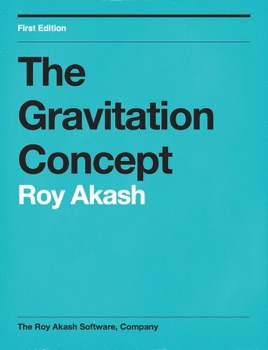## Publisher Description

In Physics, a gravitational field is a model used to explain the influence that a massive body extends into the space around itself, producing a force on another massive body. Thus, a gravitational field is used to explain gravitational phenomena, and is measured in newtons per kilogram (N/kg). In its original concept, gravity was a force between point masses. Following Isaac Newton, Pierre-Simon Laplace attempted to model gravity as some kind of radiation field or fluid, and since the 19th century explanations for gravity have usually been taught in terms of a field model, rather than a point attraction.

In a field model, rather than two particles attracting each other, the particles distort space-time via their mass, and this distortion is what is perceived and measured as a "force". In such a model one states that matter moves in certain ways in response to the curvature of space-time, and that there is either no gravitational force, or that gravity is a fictitious force.